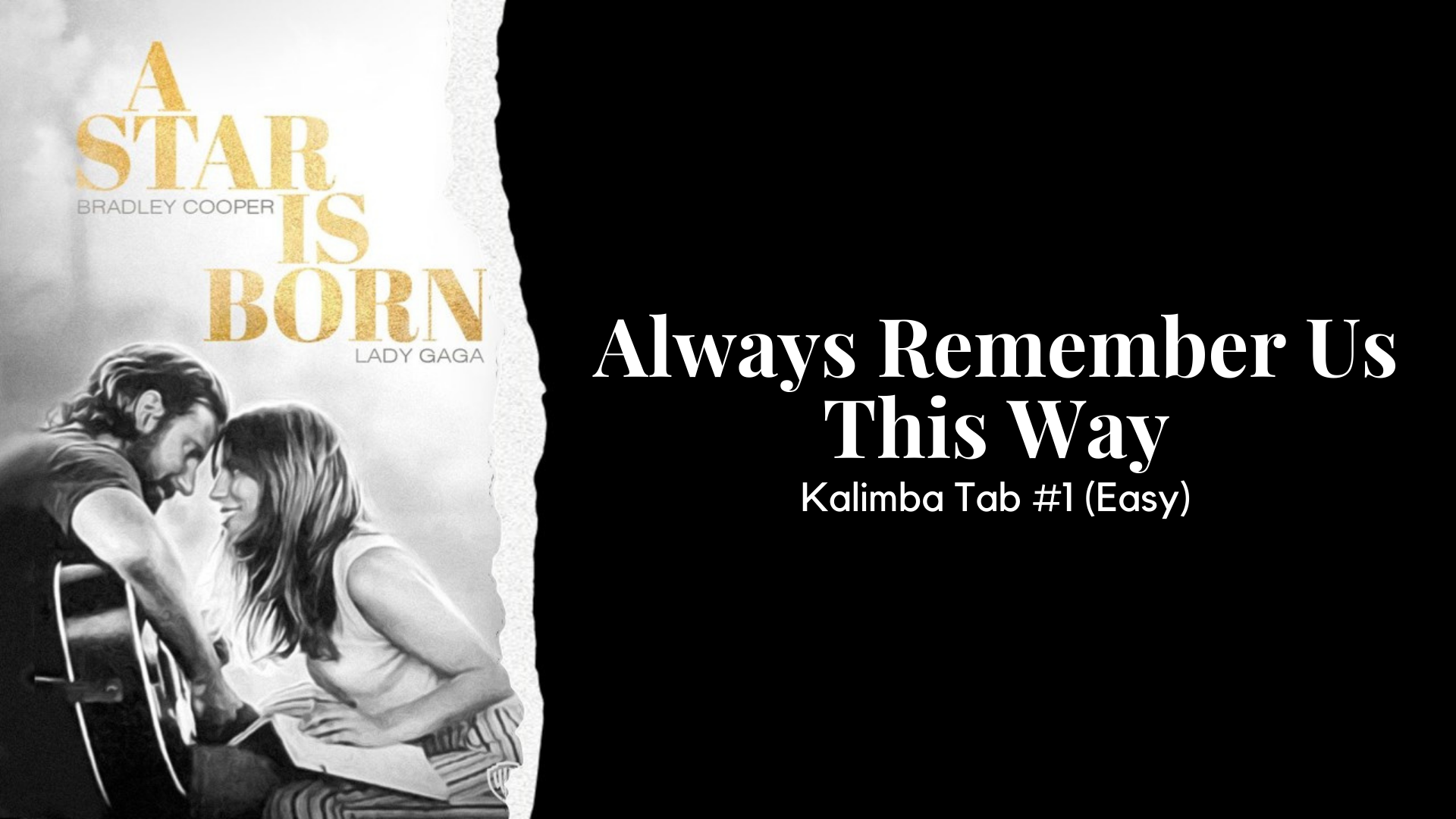White notes are the main notes

Bolded notes are harmony

Brackets mean play at the same time (A' A)

Notes with underlines are to slide across the tines (C' C E G)

G       D’ E’ D’ C’       A

That     Arizona         sky

D’ E’     D’    C’      A G

G’  (C’ C)  G  (C’ E)   G       C’

You  look   at    me  and, babe,

G     C’ G    C’        D’      B

I   wanna  catch    on     fire

G     D’ E’   D’  C’  (A E)

It's   buried   in   my   soul

D’        E’ D’ C’ (A C)          G

like       California            gold

C’    (C’ C)    G   (C’ E)  G   (C’ C

You   found  the  light     in     me

G     C’     G       C’ D’        B

that   I     couldn't   find

E’      E’       G’    (A’ E’)        A’          A’

So   when    I'm      all       choked   up

B’      (C’’ E’)     C’’       C’’    E’        G’

But         I        can't   find  the   words

E’ G’    (A’ E’)  A’       A’      B’ (C’’ E’)

Every   time   we    say      goodbye

C’’ C’’    E’ E’          D’      C’ B G

Baby,       it          hurts

E’ G’    (A’ A)  G’       E’        E’

When    the   sun   goes   down

C’        A       C’      D’ E’ E’       G’

And    the   band     won't      play

E’    (F’ F) E’ D’   C’ (B D) A     B      C’       C’

I'll      always        remember    us    this   way

D’ E’    D’   C’    (A E)

Lovers   in   the   night

D’ E’    D’ C’   (A E)     G

Poets   tryin'     to     write

G    (C’ C)    G     (C’ E)  G    (C’ C)

We   don't   know   how   to   rhyme

G        C’     G C’   D’    B

But,   damn,            we   try

C’    C’’  B’   A’ G’    (A’ A)   F G A (C’ G) A G F

But   all   I   really   know

(C’ C)      C’’     B’     A’   G’     A’   G’ F’

You're    where   I     wanna      go

G’   (C’’ C’)   G’   (C’’ E’)    G’     (C’’ C’

The    part     of      me     that's    you

G’      C’’ D’’   (B’ G’)

will    never       die

E’       E’      G’   (A’ E’)      A’         A’

So   when   I'm      all      choked   up

B’    (C’’ E’)     C’’        C’’     E’        G’     A’

But        I         can't    find   the    words

F G A (C' G) A G F

E’ G’   (A’ E’)   A’     A’   B’ (C’’ E’)

Every   time   we   say   goodbye

C’’ C’’     E’   E’   D’    C’ B G

Baby,     it        hurts

E’       G’    (A’ C’)     G’        E’      E’

When   the    sun      goes   down

C’     A     (C’ G)     D’     E’   E’ G’

And   the   band   won't   play

E’     (F’ F)      E’ D’ C’   (B D)  A    B     C’ C’

I'll   always   remember   us   this    way

E’      G’   (A’ E’)   G’ E’ E’ D’

When   you   look     at   me

C’      A     (C’ A)     D’       E’ E’   G’